# 一、简介

Transform模型是与RNN和CNN都完全不同的思路。相比Transformer，RNN/CNN的问题：

• RNN序列化处理效率提不上去。理论上，RNN效果上问题不大。
• CNN感受野小。CNN只考虑卷积核大小区域，核内参数共享，并行/计算效率不是问题，但受限于核的大小，不能考虑整个上下文。

# 二、模型结构

## 2.1 整体结构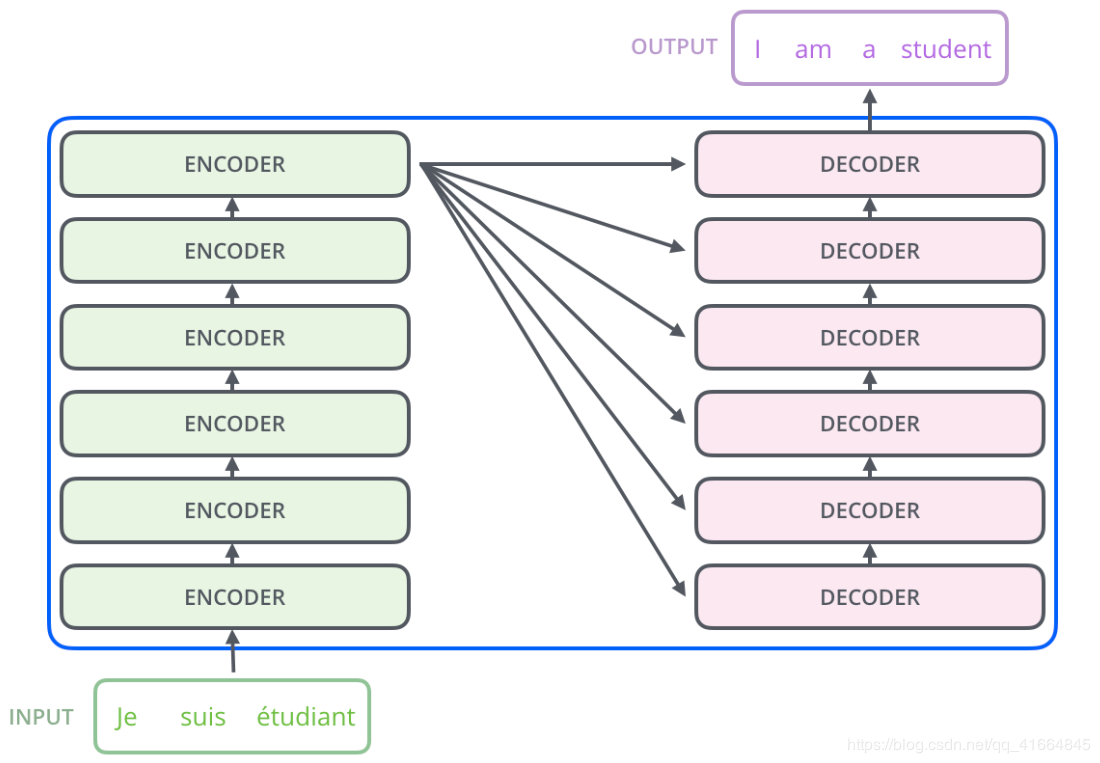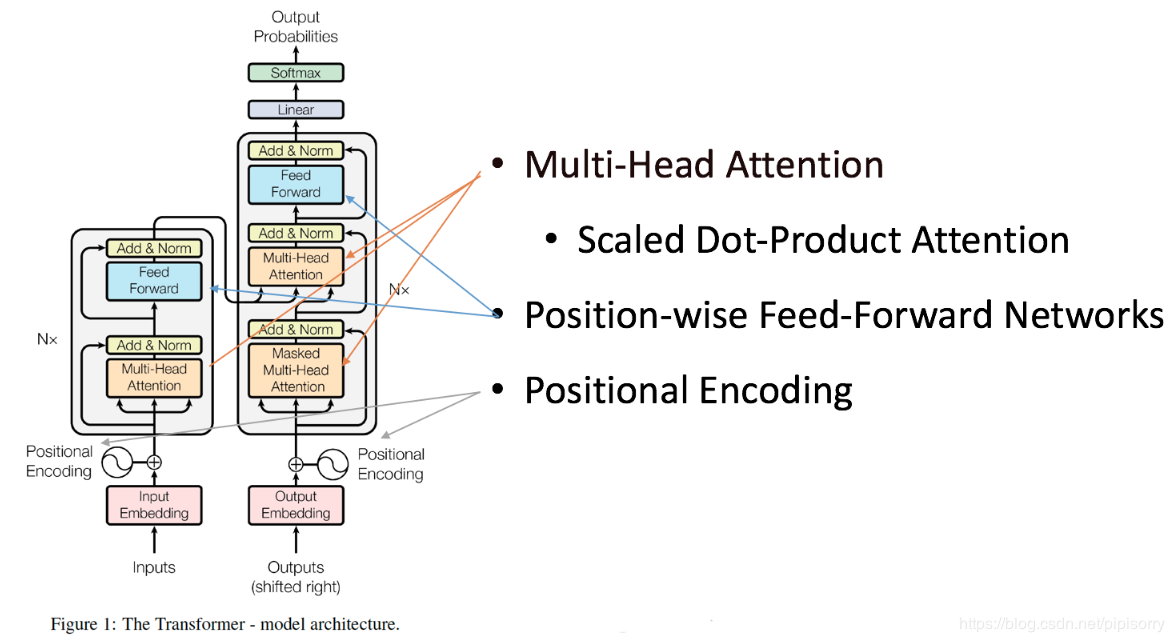Encoder由$N=6$个相同的layer组成，每一个layer就是上图左侧的单元，最左边有个$“N”$参数，这里$N=6$。每个Layer由两个sub-layer组成，分别是multi-head self-attention mechanism(左图中橙色部分)和fully connected feed-forward network(左图中蓝色部分)。其中每个sub-layer都加了residual connection和normalisation，因此可以将sub-layer的输出表示为：$sub\_layer\_output=LayerNorm(x+(SubLayer(x)))$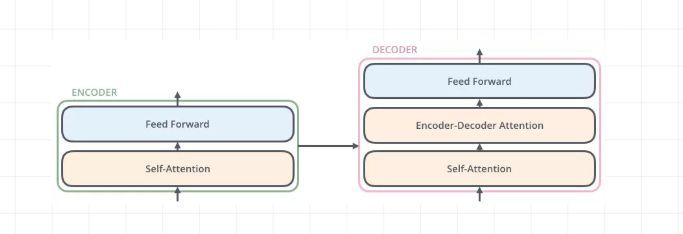## 2.2 Transformer的编解码器

### 2.2.1 编码器

• 编码器由6个相同的层堆叠在一起，每一层又有两个支层。第一个支层是一个多头的自注意机制，第二个支层是一个简单的全连接前馈网络。在两个支层外面都添加了一个residual的连接，然后进行了layer nomalization的操作。模型所有的支层以及embedding层的输出维度都是$d_{model}$

### 2.2.2 解码器

• 解码器也是堆叠了六个相同的层。不过每层除了编码器中那两个支层，解码器还加入了第三个支层对编码器栈的输出实行“多头”的Attention，如图中所示同样也用了residual以及layer normalization

• 另一个则是从Encoder到Decoder的注意力机制，而且是Decoder内部先做一次attention后再接收Encoder的输出。

Decoder有N(默认是6)层，每层包括三个sub-layers（自下往上）:

• 第一个是Masked multi-head self-attention，也是计算输入的self-attention，但是因为是生成过程，因此在时刻$i$ 的时候，大于 $i$ 的时刻都没有结果，只有小于 $i$ 的时刻有结果，因此需要做Mask，也就是去上一次的decoder层做输入。
• 第三个sub-layer是全连接网络，与Encoder相同。

• 输入：encoder的输出 和 对应$x_{i-1}$位置decoder的输出。所以中间的attention不是self-attention，它的$K，V$来自encoder，$Q$来自上一位置decoder的输出。向量$K$（键向量）和$V$（值向量）的注意力向量集来自 Encoder。这些向量将被每个解码器用于自身的“编码-解码注意力层”，并作用在Decoder的每一层。

• 解码：这里要特别注意一下，编码可以并行计算，一次性全部encoding出来，但解码不是一次把所有序列解出来的，而是像RNN一样一个一个解出来的，因为要用上一个位置的输入当作attention的query

• 输出：对应输入$x_i$的输出词的概率分布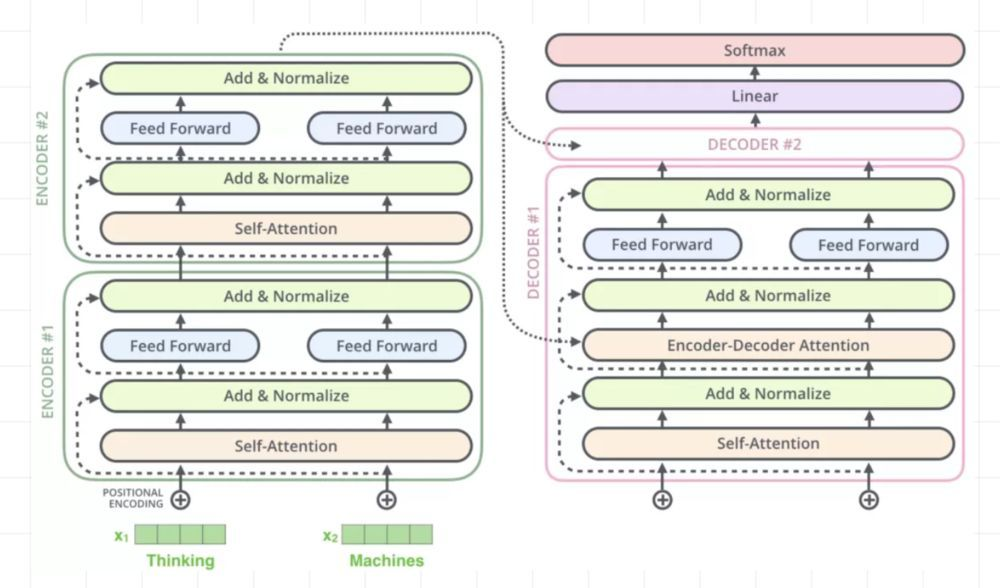## 2.3 输入层

class Embeddings(nn.Module):
def __init__(self, d_model, vocab):
super(Embeddings, self).__init__()
self.lut = nn.Embedding(vocab, d_model)
self.d_model = d_model

def forward(self, x):
return self.lut(x) * math.sqrt(self.d_model)


## 2.4 位置向量

$PE_{(pos,2i)}=sin(pos/10000^{2i/d_{\text{model}}})$

$PE_{(pos,2i+1)} = cos(pos / 10000^{2i/d_{\text{model}}})$

## 2.5 Attention模型

### 2.5.1 NLP中的注意力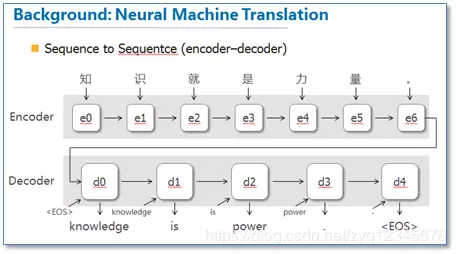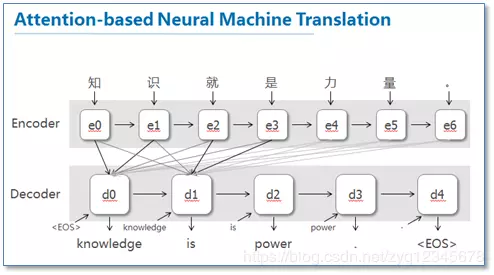NLP中有个非常常见的一个三元组概念：Query、Key、Value，其中绝大部分情况Key=Value。在机器翻译中，Query是已经翻译出来的部分，Key和Value是源语言中每个词的表达（编码/向量），没有注意力时直接拿Query就去预测下一个词，注意力机制的计算就是用Query和Key计算出一组权重，赋权到Value上，拿Value去预测下一词。### 2.5.2 Scaled attention

$Attention(Q,K,T) = soft\max (\frac{{Q{K^T}}}{{\sqrt {{d_k}} }})V$attention可视化的效果（这里不同颜色代表attention不同头的结果，颜色越深attention值越大）。可以看到self-attention在这里可以学习到句子内部长距离依赖"making…….more difficult"这个短语。1. $QK^T$Query向量和Key向量做点积2. scale放缩、softmax归一化、dropout随机失活/置零
3. 将权重矩阵加权到Value上，维度未变化。### 2.5.3 多头自注意力

$MultiHead(Q,K,V) = Concat(head_1,...,head_h)$

$where: head_i = Attention(Q{W_i}^Q,K{W_i}^K,V{W_i}^V)$“拆”的过程是一个独立的(different)、可学习的(learned)线性映射。实际实现可以是$h$个全连接层，每个全连接层输入维度是$512$，输出$512/h$；也可以用一个全连接，输入输出均为$512$，输出之后再切成h份。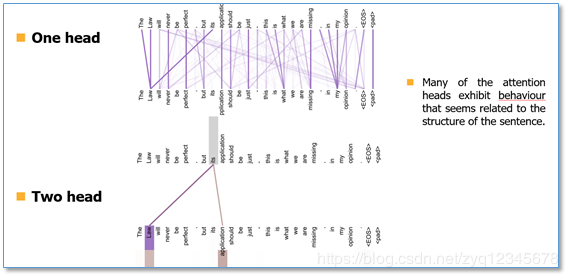Transformer以三种不同的方式使用了多头attention。

1. 在encoder-decoder的attention层，queries来自于之前的decoder层，而keys和values都来自于encoder的输出。这个类似于很多已经提出的seq2seq模型所使用的attention机制。
2. 在encoder含有self-attention层。在一个self-attention层中，所有的keys,values以及queries都来自于同一个地方，本例中即encoder之前一层的的输出。

1. 从每个编码器的输入向量（每个单词的词向量）中生成三个向量。也就是说对于每个单词，我们创造一个查询向量$Q$、一个键向量$K$和一个值向量$V$。这三个向量是通过词嵌入与三个权重矩阵后相乘创建的。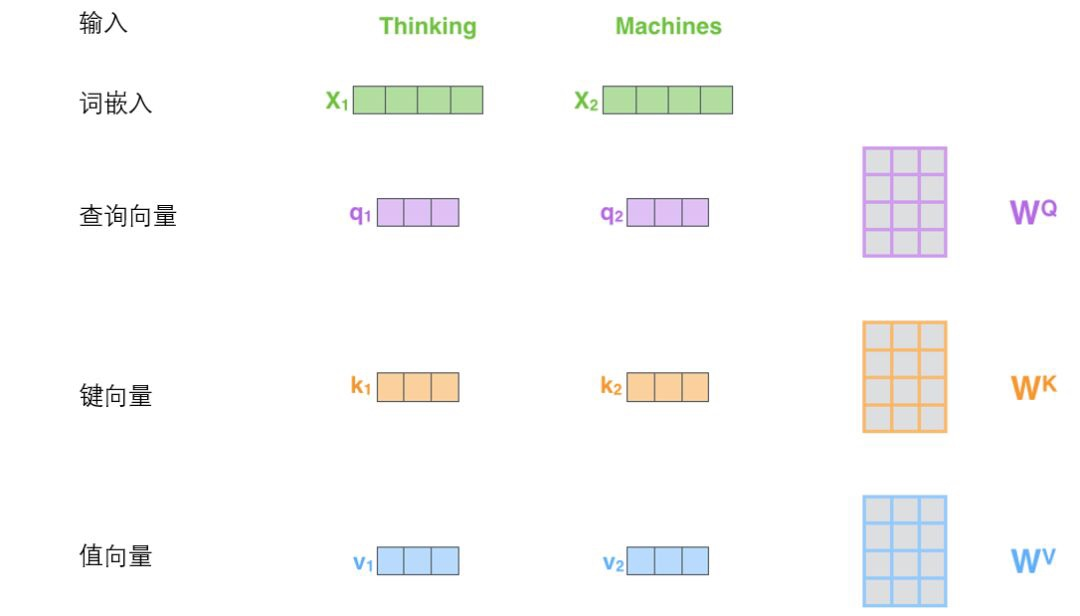其中：$\begin{array}{l} {Q_i} = {X_i} * W_i^Q\\ {K_i} = {X_i} * W_i^K\\ {V_i} = {X_i} * W_i^V \end{array}$

可以发现这些新向量在维度上比词嵌入向量更低。他们的维度是$64$，而词嵌入和编码器的输入/输出向量的维度是$512$，但实际上不强求维度更小，这只是一种基于架构上的选择，它可以使多头注意力（multiheaded attention）的大部分计算保持不变。

1. 计算得分，假设我们在为这个例子中的第一个词“Thinking”计算自注意力向量，我们需要拿输入句子中的每个单词对“Thinking”打分。这些分数决定了在编码单词“Thinking”的过程中有多重视句子的其它部分。这些分数是通过打分单词（所有输入句子的单词）的键向量$K$与“Thinking”的查询向量$Q$相点积来计算的。所以如果我们是处理位置最靠前的词的自注意力的话，第一个分数是$q_1$$k_1$ 的点积，第二个分数是$q_1$$k_2$ 的点积。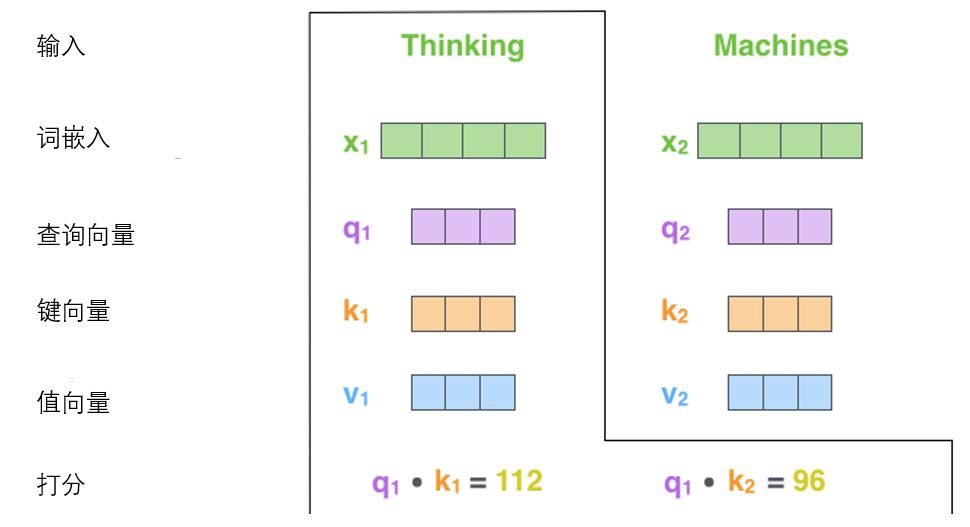2. 缩放和softmax：是将分数除以$8$($8$是论文中使用的键向量的维数$64$的平方根，这会让梯度更稳定。这里也可以使用其它值，$8$只是默认值)，然后通过softmax传递结果。softmax的作用是使所有单词的分数归一化，得到的分数都是正值且和为$1$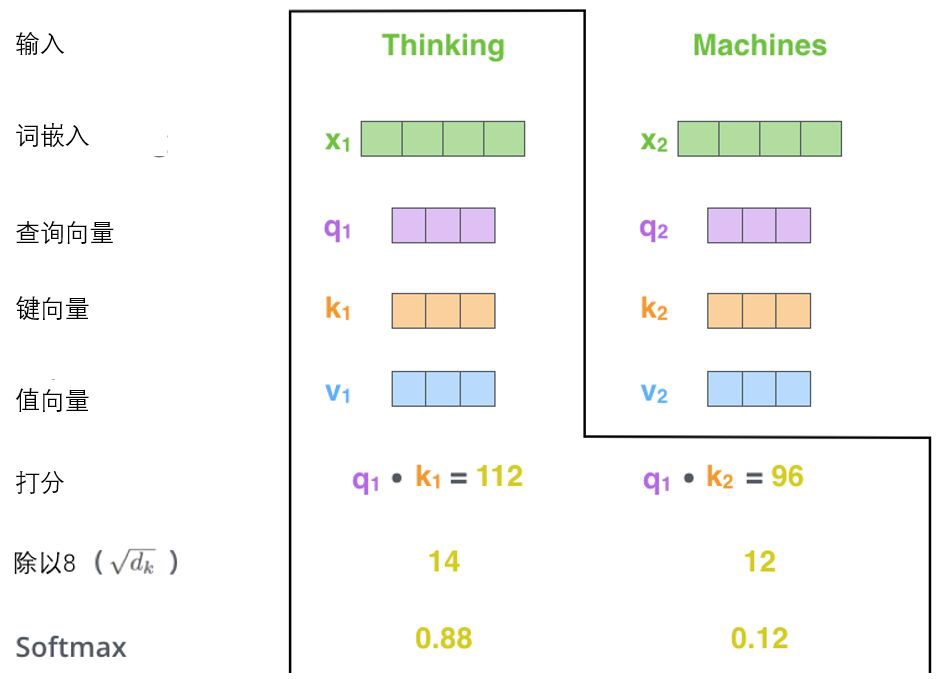这个softmax分数决定了每个单词对编码当下位置（“Thinking”）的贡献。显然，已经在这个位置上的单词将获得最高的softmax分数，但有时关注另一个与当前单词相关的单词也会有帮助。

3. 将每个值向量$V$乘以softmax分数(这是为了准备之后将它们求和)，不过这里有一个问题，比如输入有$3$个单词，就有$3$$q$$3$$k$，比如第一个单词评分分别是$q_1*k_1$$q_1*k_2$$q_1*k_3$，这里就有$3$个softmax值，这3个值分别跟对应的$v_i$相乘，就有$3$个向量。这里的直觉是希望关注语义上相关的单词，并弱化不相关的单词(例如，让它们乘以$0.001$这样的小数)。

4. 加权值向量求和，然后即得到自注意力层在该位置的输出(在我们的例子中是对于第一个单词)，也就是第4步中我们说的3个向量。自注意力的另一种解释就是在编码某个单词时，就是将所有单词的表示（值向量）进行加权求和，而权重是通过该词的表示（键向量）与被编码词表示（查询向量）的点积并通过softmax得到。不过现在仔细想想，求和是各个向量同位置上的求和还是所有向量累加？看下图就是多个向量同位置的元素相加。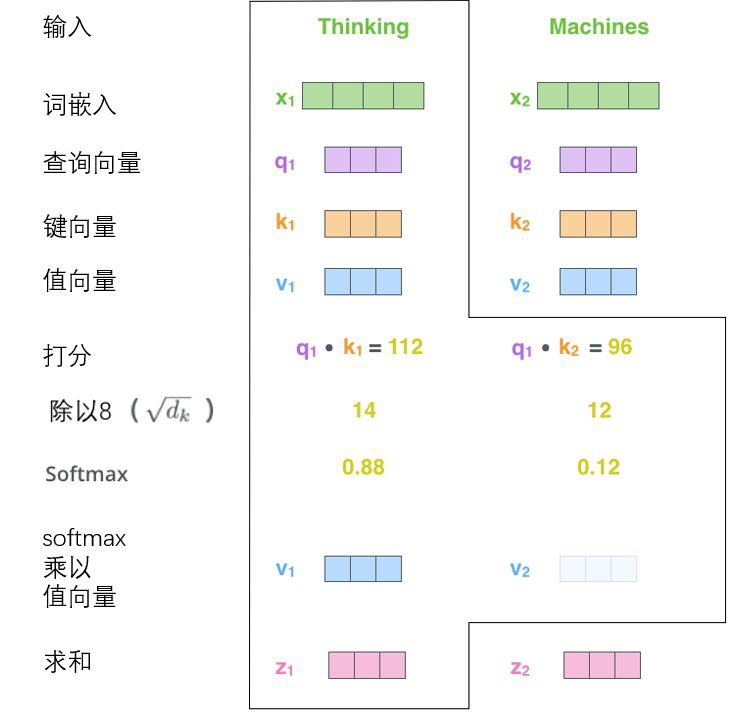• 它扩展了模型专注于不同位置的能力。在上面的例子中，虽然每个编码都在z1中有或多或少的体现，但是它可能被实际的单词本身所支配。如果我们翻译一个句子，比如“The animal didn’t cross the street because it was too tired”，我们会想知道“it”指的是哪个词，这时模型的“多头”注意机制会起到作用。
• 它给出了注意力层的多个“表示子空间”（representation subspaces）。接下来我们将看到，对于“多头”注意机制，我们有多个查询$Q$、键$K$、值$V$权重矩阵集(Transformer使用八个注意力头，因此我们对于每个编码器/解码器有八个矩阵集合)。这些集合中的每一个都是随机初始化的，在训练之后，每个集合都被用来将输入词嵌入(或来自较低编码器/解码器的向量)投影到不同的表示子空间中。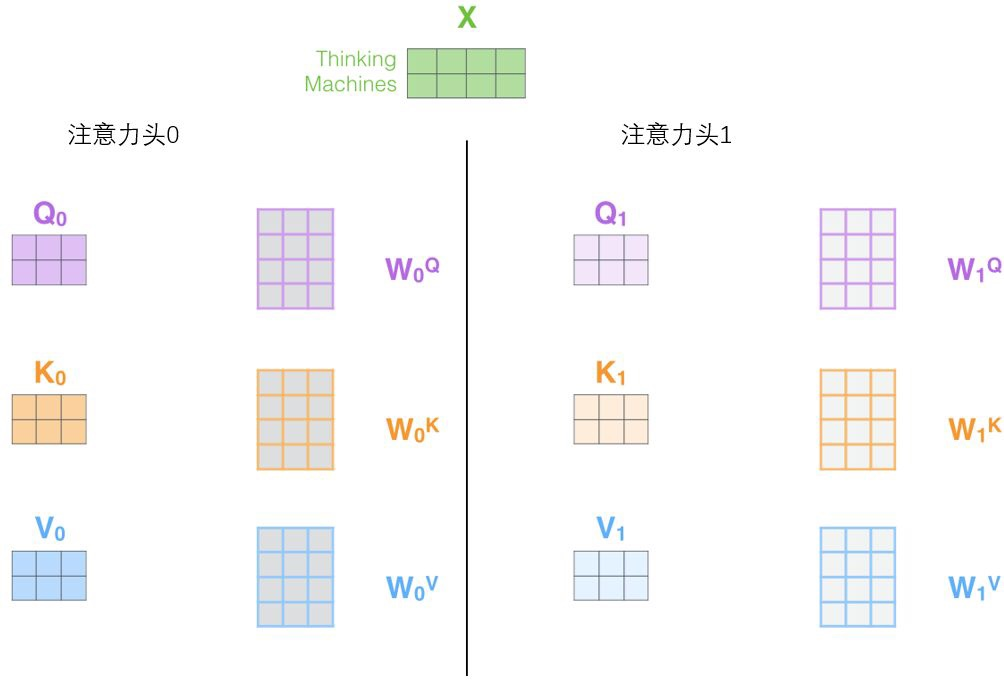multi-head attention则是通过h个不同的线性变换对$Q，K，V$进行投影，最后将不同的attention结果拼接起来：

$MultiHead(Q,K,V) = Concat(head_1,...,head_h)$

$where: head_i = Attention(Q{W_i}^Q,K{W_i}^K,V{W_i}^V)$

$head_i$公式中我们可以看出每一个$head_i$都独立维护一套$Q/K/V$的权值矩阵。假设我们有8个head，那么最终我们可以得到8个head值，然后拼接在一起作为一个向量，与$W_0$ 相乘，作为Multi-head self-attention 层的输出，计算流程如下图所示：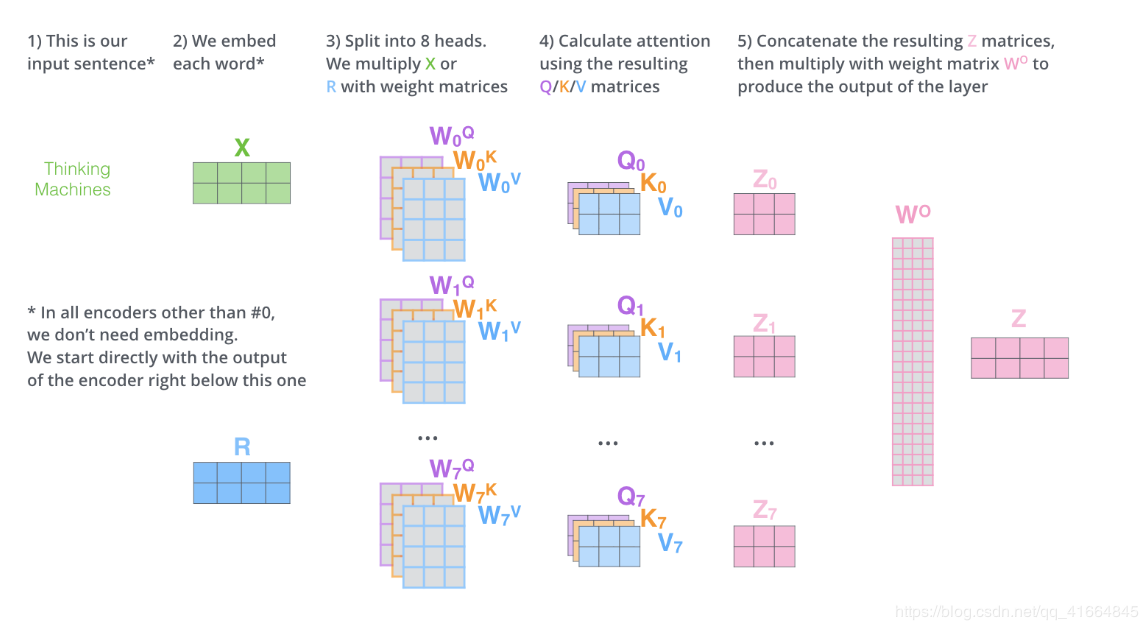self-attention则是取$Q，K，V$维度相同。文章中attention的计算采用了scaled dot-product，即：
$Attention(Q,K,T) = soft\max (\frac{{Q{K^T}}}{{\sqrt {{d_k}} }})V$

## 2.6 Position-wise feed-forward networks(位置全链接前馈网络)

$FFN(x) = max(0,xW_1 + b_1)W_2 + b_2$

• Position-wise: 顾名思义，就是对每个position采用相同的操作。
• Feed-Forward Network: 就是最普通的全连接神经网络，这里采用的两层，relu作为激活函数。

Position-wise feed forward network其实就是一个MLP 网络，每个 $d_model$ 维向量 $x$ 在此先由 $xW_1+b_1$ 变为 $d_f$ 维的 $x’$，再经过$max(0,x′)W_2+b_2$回归$d_{model}$ 维。hidden_size变化为：$768->3072->768（或者512->2048->512）$。之后就是对hidden层进行dropout，最后加一个resnetnormalization（tensor的最后一维，即feature维进行）。Transformer通过对输入的文本不断进行这样的注意力机制层和普通的非线性层交叠来得到最终的文本表达。

## 2.7 残差连接网络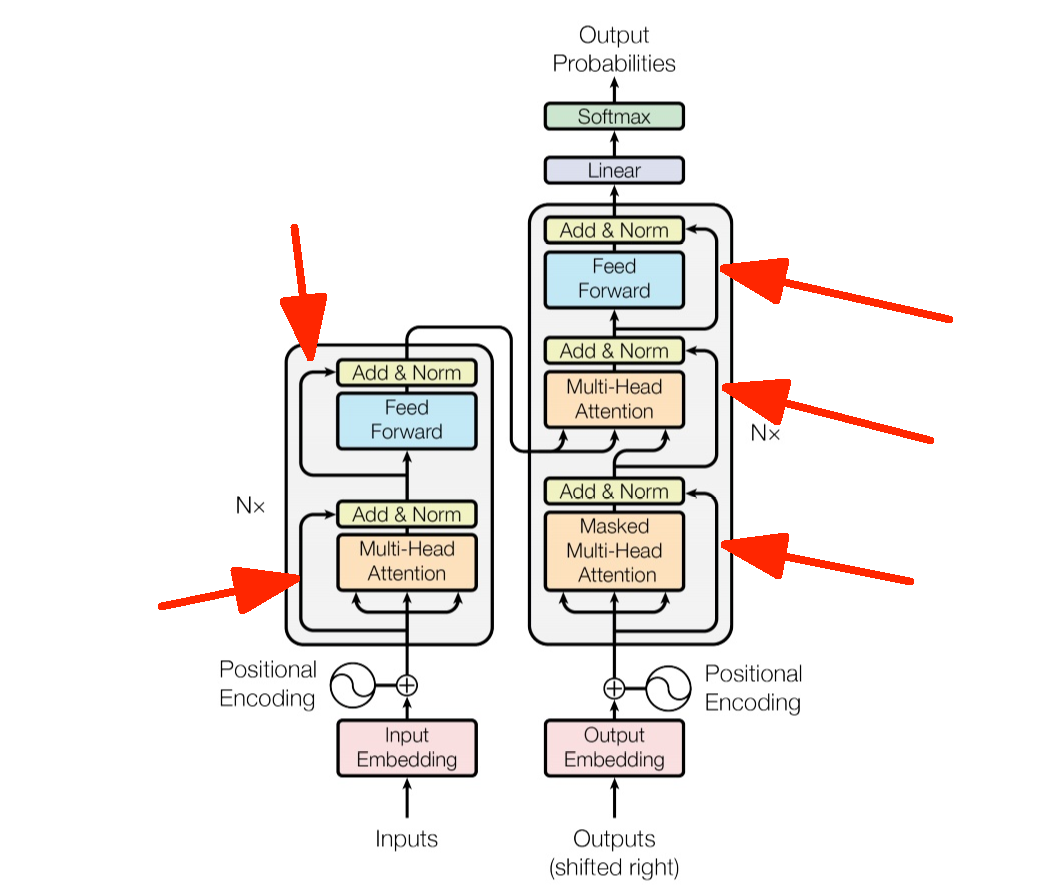2. sequence mask在上文中我们提到，预测$t$时刻的输出值$y_t$，应该使用全部的输入序列$X$，和$t$时刻之前的输出序列$(y_1,y_2,……,y_{t-1})$进行预测。所以在训练时，应该将$t-1$时刻之后的信息全部隐藏掉。所以需要用到sequence mask。实现也很简单，就是用一个上三角矩阵，上三角值均为1，下三角值均为0，对角线值为0，与输入序列相乘，就达到了目的。

# 三、总结

## 3.2 模型不足

• 粗暴的抛弃RNN和CNN虽然非常炫技，但是它也使模型丧失了捕捉局部特征的能力，RNN + CNN + Transformer的结合可能会带来更好的效果。

• Transformer失去的位置信息其实在NLP中非常重要，而论文中在特征向量中加入Position Embedding也只是一个权宜之计，并没有改变Transformer结构上的固有缺陷。

# 四、参考

©️2019 CSDN 皮肤主题: 大白 设计师: CSDN官方博客